## Tamilnadu Samacheer Kalvi 9th Maths Solutions Chapter 5 Coordinate Geometry Ex 5.3

9th Maths Exercise 5.3 Solution Question 1.
Find the mid-points of the line segment joining the points
(i) (-2, 3) and (-6, -5)
(ii) (8, -2) and (-8, 0)
(iii) (a, b) and (a + 2b, 2a – b)
(iv) $$\left(\frac{1}{2},-\frac{3}{7}\right)$$ and $$\left(\frac{3}{2}, \frac{-11}{7}\right)$$
Solution: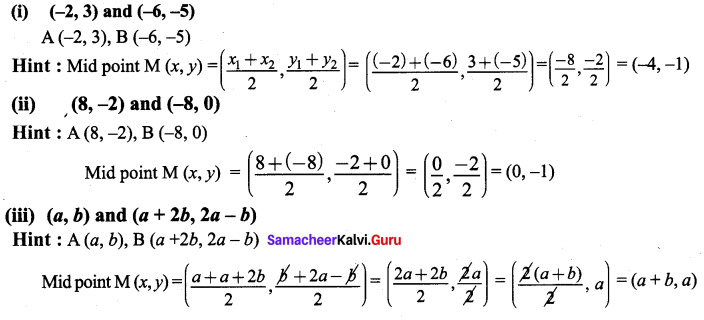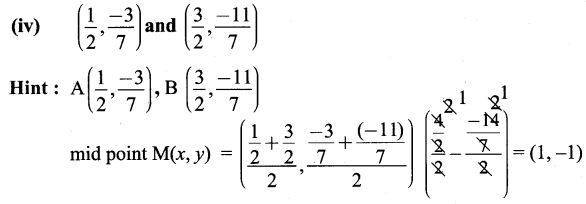9th Maths Exercise 5.3 Question 2.
The centre of a circle is (-4, 2). If one end of the diameter of the circle is (-3, 7) then find the other end.
Solution: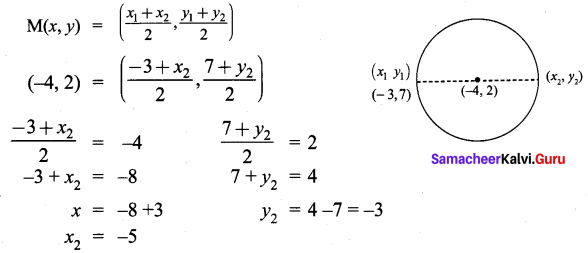∴ The other end is (-5, -3)

9th Maths Exercise 5.3 Samacheer Kalvi Question 3.
If the mid-point (x, y) of the line joining (3, 4) and (p, 7) lies on 2x + 2y + 1 = 0, then
what will be the value of p?
Solution: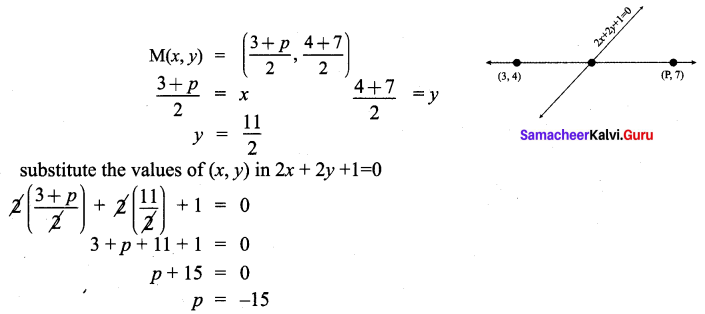9th Maths 5.3 Question 4.
The midpoint of the sides of a triangle are (2, 4), (-2, 3) and (5, 2). Find the coordinates of the vertices of the triangle.
Solution: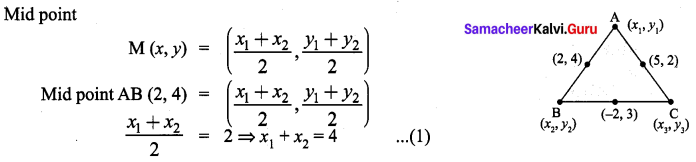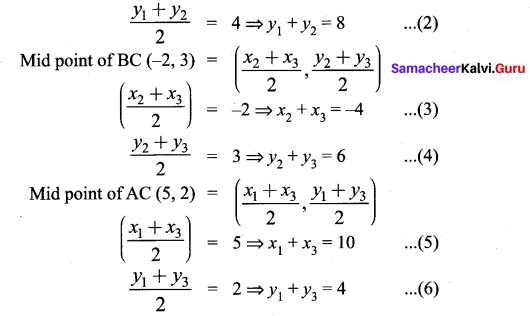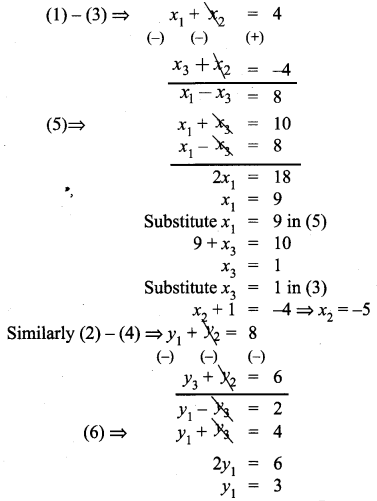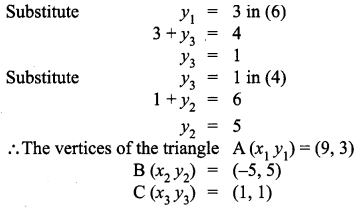Ex 5.3 Class 9 Samacheer Question 5.
O(0, 0) is the centre of a circle whose one chord is AB, where the points A and B are (8, 6) and (10, 0) respectively. OD is the perpendicular from the centre to the chord AB. Find the coordinates of the mid-point of OD.
Solution: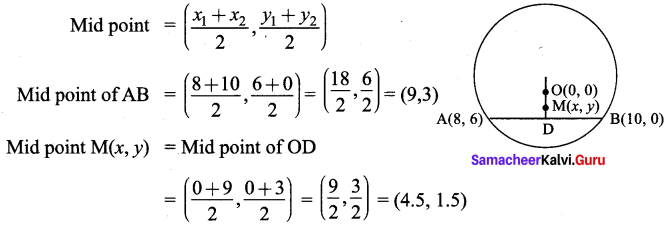Coordinate Geometry Class 9 Exercise 5.3 Question 6.
The points A (-5, 4), B (-1, -2) and C(5, 2) are the vertices of an isosceles right¬angled triangle where the right angle is at B. Find the coordinates of D so that ABCD is a square.
Solution: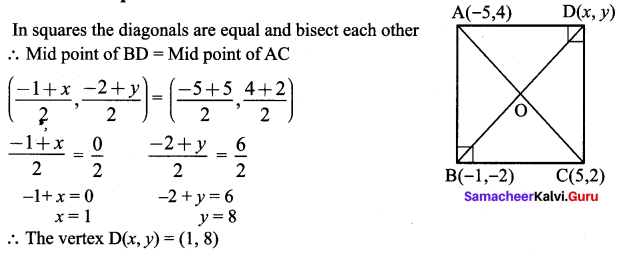Class 9 Maths Exercise 5.3 Solution Question 7.
The points A(-3, 6), B(0, 7) and C(1, 9) are the mid points of the sides DE, EF and FD of a triangle DEF. Show that the quadrilateral ABCD is a parallelogram.
Solution:
In a parallelogram diagonals bisect each other and diagonals are not equal.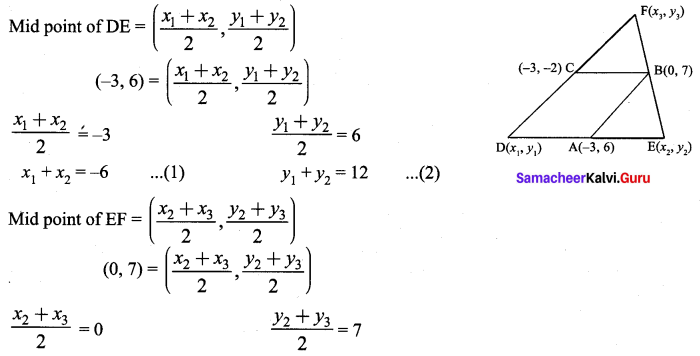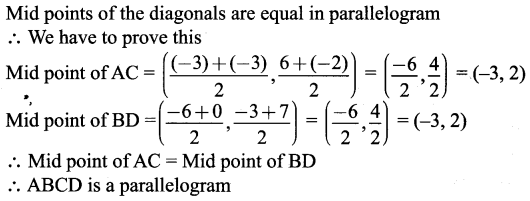Exercise 5.3 Class 10 Samacheer Kalvi Question 8.
A (-3, 2), B (3, 2) and C(-3, -2)are the vertices of the right triangle, right angled at A. Show that the mid point of the hypotenuse is equidistant from the vertices.
Solution: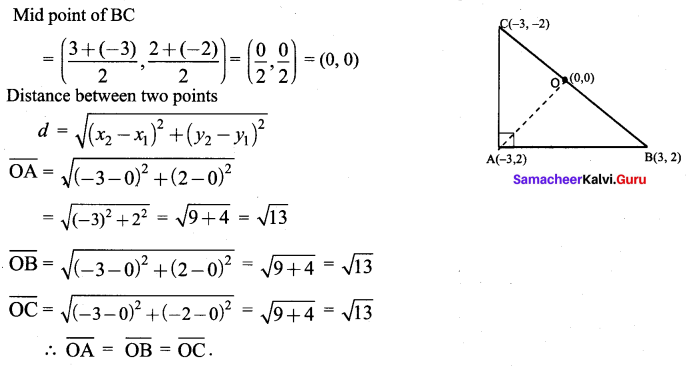Hence proved